# Image Manipulations in OpenCV (Part-2)

Published  January 31, 2019   0
M
Author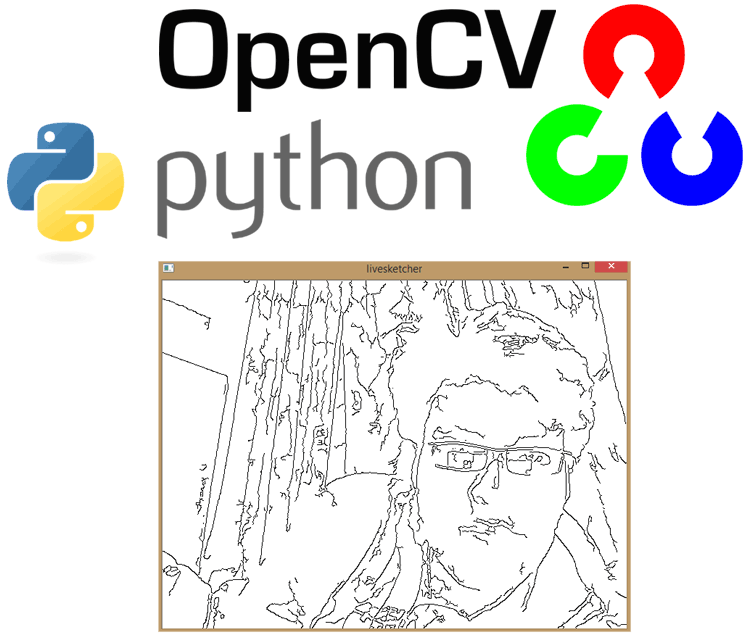In the previous tutorials, we have learned about OpenCV and done some basic image processing and then in next tutorial we have done some image manipulation in OpenCV like cropping, rotation, image transformation etc. So in continuation with previous Image Manipulation tutorial, here we learn some more image manipulation techniques like and at the end of the tutorial we will build a python-opencv program to make live sketch from the webcam live feed. This application will use many of Image processing functions which we have learned so far or will learn in this tutorial, so this will be a good practical example to cover all the functions.

As told in the previous tutorial, OpenCV is Open Source Commuter Vision Library which has C++, Python and Java interfaces and supports Windows, Linux, Mac OS, iOS and Android. So it can be easily installed in Raspberry Pi with Python and Linux environment. And Raspberry Pi with OpenCV and attached camera can be used to create many real-time image processing applications like Face detection, face lock, object trackingcar number plate detectionHome security system etc.

In this tutorial, we are going to see some more image manipulations using Python OpenCV. Here we will learn to apply the following function on an image using Python OpenCV:

• Convolution & Blurring
• Sharpening - Reversing the image blurs
• Thresholding (Binarization)
• Dilation, Erosion, Opening/Closing
• Edge detection and Image gradients
• Perspective & Affine Transform
• Live Sketch Application

### 1. Bitwise Operations and Masking

Making a square

```import cv2
import numpy as np
#we use only two dimensions because this is a grayscale image, if we were using a
#colored image, we had then used a rectangle=np.zeros((300,300,3),np.uint8)
#Making a square
square =np.zeros((300,300),np.uint8)
cv2.rectangle(square,(50,50),(250,250),255,-1)
cv2.imshow("square",square)
cv2.waitKey(0)```

Making an ellipse

```ellipse=np.zeros((300,300),np.uint8)
cv2.ellipse(ellipse,(150,150),(150,150),30,0,180,255,-1)
cv2.imshow("ellipse",ellipse)
cv2.waitKey(0)```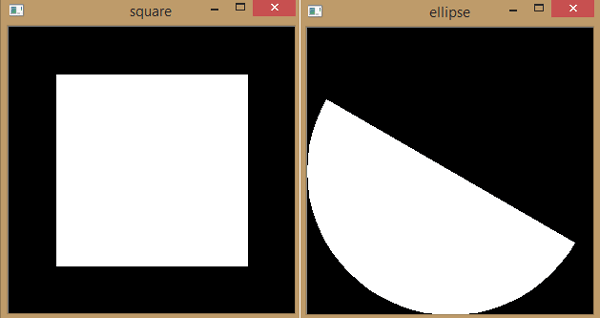Experimenting with bitwise operations

#remember the two shapes to be masked must be of same dimensions, that’s why initially we have created a canvas of 300x300

#AND_shows only where the two intersect

```BitwiseAND=cv2.bitwise_and(square,ellipse)
cv2.imshow("AND",BitwiseAND)
cv2.waitKey(0)     ```

#OR_shows only where either square or ellipse is

```BitwiseOR=cv2.bitwise_or(square,ellipse)
cv2.imshow("OR",BitwiseOR)
cv2.waitKey(0)

```

#XOR_shows only where either exists by itself

```BitwiseXOR=cv2.bitwise_xor(square,ellipse)
cv2.imshow("XOR",BitwiseXOR)
cv2.waitKey(0)```

#NOT_shows everything that’s not part of the ellipse and NOT operation can be applied only to single figure

```BitwiseNOT_elp=cv2.bitwise_not(ellipse)
cv2.imshow("NOT_ellipse",BitwiseNOT_elp)
cv2.waitKey(0)
cv2.destroyAllWindows()```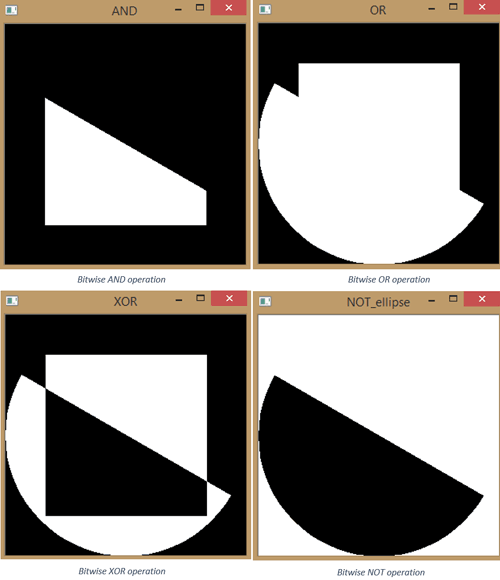### 2. Convolution & Blurring

A convolution is an mathematical operation performed on two functions producing a third function which is typically a modified version of original function.

Output image = image  FunctionKernel size

In computer vision we use kernel’s to specify the size over which we run our manipulating function over our image.

Blurring is an operation where we average the pixels within a region(Kernel)

OpenCV blurs an image by applying kernels, a kernel tells you how to change the value of any given pixel by combining it with different amount of neighboring pixels the kernel is applied to every pixel in the image one by one to produce the final image.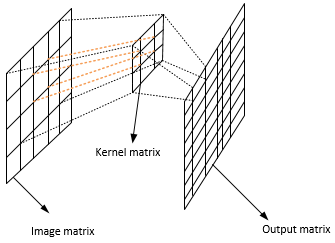Simply saying, an image convolution is simply an element wise multiplication of two matrices followed by a sum.

We can simply understand it by the following example.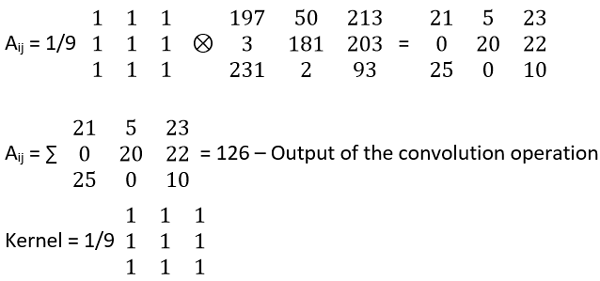The above is a 3X3 Kernel.

We multiply by 1/25 to normalize i.e. sum to 1 we had been increasing the intensity or decreasing the intensity as in the case of brightening or darkening of images.

Let’s test a opencv blurring method filter2D, given by the function cv2.filter2D (image, -1, kernel)

```import cv2
import numpy as np
cv2.imshow('original',image)
cv2.waitKey(0)```

#creating a 3x3 kernel matrix

`kernel_3x3=np.ones((3,3),np.float32)/9`

# we use cv2.filter2D to convolve the kernel with an image

```blurred=cv2.filter2D(image,-1,kernel_3x3)
cv2.imshow('3x3_blurring', blurred)
cv2.waitKey(0) ```

#creating a 7x7 kernel matrix

`kernel_7x7=np.ones((7,7),np.float32)/49`

# we use cv2.filter2D to convolve the kernel with an image

```blurred=cv2.filter2D(image,-1,kernel_7x7)
cv2.imshow('7x7_blurring', blurred)
cv2.waitKey(0)
cv2.destroyAllWindows()```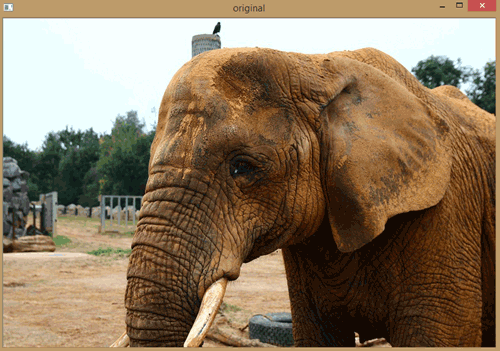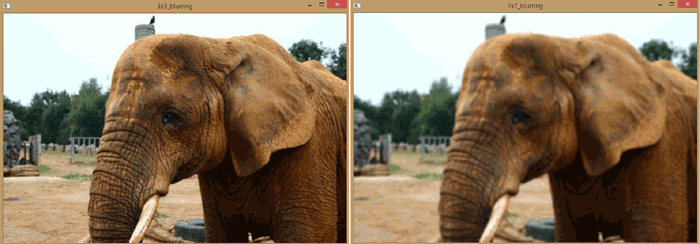There are other types of blurring methods too:

cv2.blur – Averages value over a specified window.

cv2.GaussianBlur – Similar but uses a Gaussian window (more emphasis on points around the center).

cv2.medianBlur– Uses median of all elements in the window.

cv2.bilateralFilter– Blurs while keeping the edges sharp, it preserves the edges and line details.

We will see one by one below, first display the original image using below code:

```import cv2
import numpy as np
cv2.imshow('original', image)
cv2.waitKey(0)```

cv2.blur:

In this method averaging is done by convolving the image with a normalized box filter, this takes the place under the box and replaces the central element. Here the box size needs to be odd and positive.

```#cv2.blur
blur=cv2.blur(image,(3,3))
cv2.imshow('Averaging', blur)
cv2.waitKey(0)```

cv2.GaussianBlur:

```#cv2.GaussianBlur
#instead of box filter, let’s try Gaussian kernel
Gaussian=cv2.GaussianBlur(image,(7,7),0)
cv2.imshow('Gaussian blurring',Gaussian)
cv2.waitKey(0)```

cv2.medianBlur:

It takes median of all pixels under the kernel area and central element is replaced with this median value.

```#cv2.medianBlur
#takes median of all pixels under the kernel area and central element
#is replaced with this median value.
median=cv2.medianBlur(image,5)​
cv2.imshow('median blurring',median)
cv2.waitKey(0)```

cv2.bilateralFilter:

Bilateral is very effective in noise removal while keeping the edges sharp

```#cv2.bilateralFilter
#Bilateral is very effective in noise removal while keeping the edges sharp
bilateral=cv2.bilateralFilter(image,9,75,75)
cv2.imshow('bilateral blurring',bilateral)
cv2.waitKey(0)
cv2.destroyAllWindows()```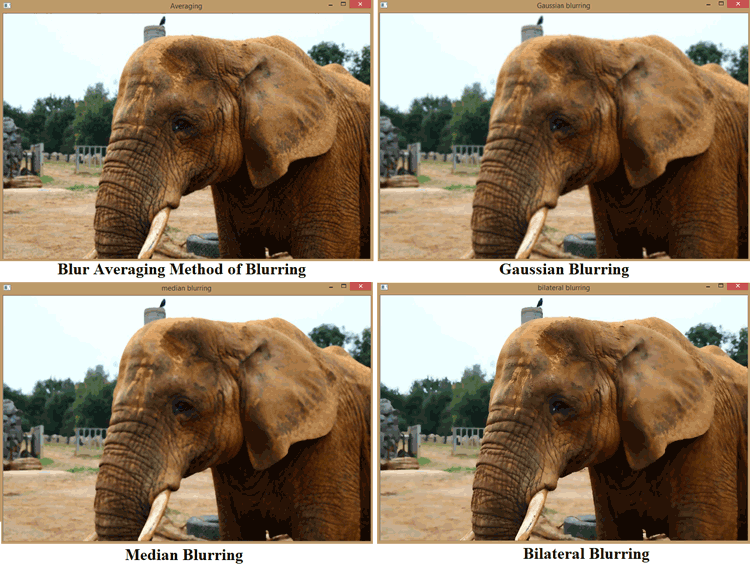Image De-noising-non Local means Denoising

```import cv2
import numpy as np
cv2.imshow('original',image)
cv2.waitKey(0)```

```#parameter after None is the filter strength 'h'(5-10 is a good range)
#next is h for color components, set as same value as h again```

```dst=cv2.fastNlMeansDenoisingColored(image,None,6,6,7,21)
cv2.imshow('Fast means denois',dst)
cv2.waitKey(0)
cv2.destroyAllWindows()```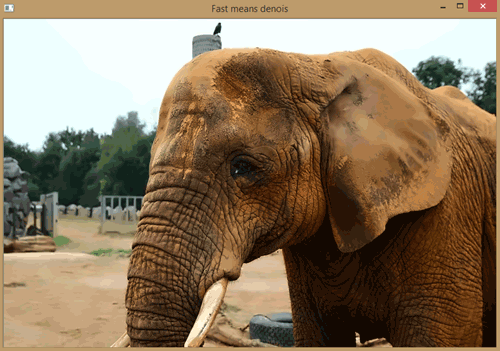There are 4 variations of non-local means denoising

cv2.fastNlMeansDenoising() – for single gray scale image

cv2.fastNlMeansDenoisingColored() – Single color image

cv2.fastNlmeansDenoisingMulti() – for image sequence grayscale

cv2.fastNlmeansDenoisingcoloredMulti() – for image sequence colored

### 3. Sharpening - Reversing the image blurs

Sharpening is the opposite of blurring, it strengths or emphasizes on edges in the image.

```Kernel = [-1,-1,-1],

[-1, 9,-1],

[-1,-1,-1]```

Our kernel matrix sums up to one, so there is no need to normalize (i.e. multiply by a factor to same brightness as of original), if kernel is not normalized to 1 the image would be brighter or darker.

```import cv2
import numpy as np
cv2.imshow('original',image)
cv2.waitKey(0)```

kernel_sharpening=np.array([[-1,-1,-1],

[-1, 9,-1],

[-1,-1,-1]])

#applying sharpening kernel to input image

```sharpened=cv2.filter2D(image,-1,kernel_sharpening)
cv2.imshow('sharpened image',sharpened)
cv2.waitKey(0)
cv2.destroyAllWindows()```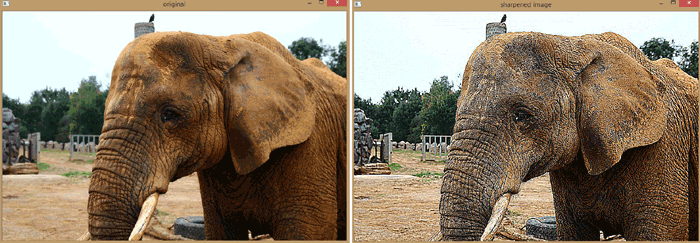### 4. Threshoding (Binarization)

Thresholding is act of converting an image into binary form. In opencv there is separate function for thresholding defined as

Cv2.threshold(image, threshold value, Max value, threshold type)

There are following threshold types:

• cv2.THRESH_BINARY –  most common
• cv2. THRESH_BINARY_INV – most common
• cv2.THRESH_TRUNC
• cv2.THRESH_TOZERO
• cv2. THRESH_TOZERO_INV

NOTE: image is needed to be converted to grayscale before thresholding

```import cv2
import numpy as np
cv2.imshow('original',image)
cv2.waitKey(0)```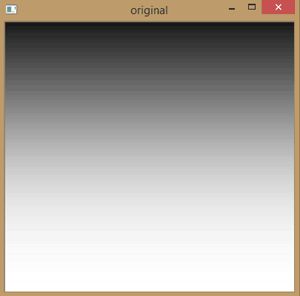#value below 127 go to 0 (black), and above 127 goes to 255(white)

```_,thresh1=cv2.threshold(image,127,255,cv2.THRESH_BINARY)
cv2.imshow('1 threshold',thresh1)
cv2.waitKey(0)

```

#value below 127 goes to 255 and values above 127 goes to 0(reverse of above)

```_,thresh2=cv2.threshold(image,127,255,cv2.THRESH_BINARY_INV)
cv2.imshow('2 threshold',thresh2)
cv2.waitKey(0)```

#value above 127 are truncated (held) at 127, the 255 argument is unused.

```_,thresh3=cv2.threshold(image,127,255,cv2.THRESH_TRUNC)
cv2.imshow('3 thresh trunc', thresh3)
cv2.waitKey(0)```

#values below 127 goes to 0, above 127 are unchanged

``` _,thresh4=cv2.threshold(image,127,255,cv2.THRESH_TOZERO)
cv2.imshow('4 threshold', thresh4)
cv2.waitKey(0)```

#Revesrse of above, below 127 is unchanged, above 127 goes to zero

```_,thresh5=cv2.threshold(image,127,255,cv2.THRESH_TOZERO_INV)
cv2.imshow('5 threshold', thresh5)
cv2.waitKey(0)
cv2.destroyAllWindows()```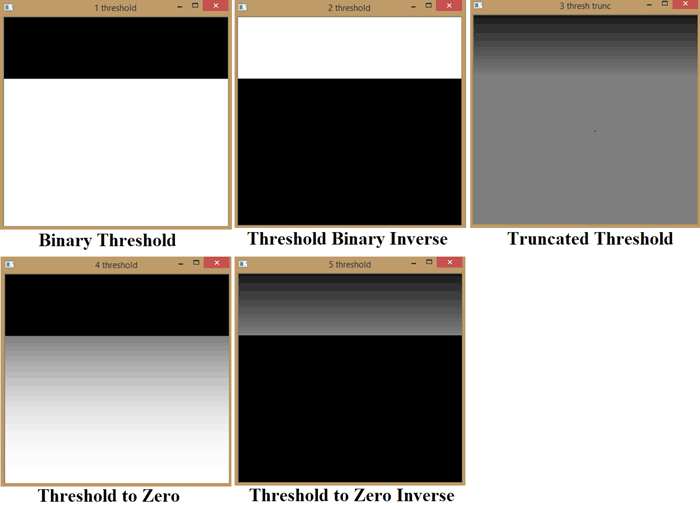### 5. Dilation, Erosion, Opening/Closing

These are the operations in the field of mathematical morphology

Dilation – it adds pixels to the boundaries of object in an image.

Erosion – Removes pixels at the boundaries of object in an image.

Opening – Erosion followed by dilation.

Closing – Dilation followed by erosion.

Opening is very much helpful in denoising the images as it first thins the image by erosion (removes the noise) and then dilates it.

Confusion with dilation and erosion

There is sometimes confusion between dilation and erosion usually in pictures with white background, as opencv considers white background as image to be dilated or eroded instead of original picture, so in this case erosion works as dilation and vice-versa, as shown in image sample shown below.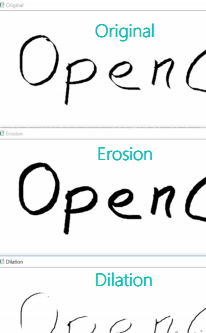Remember, Dilation adds pixels to the boundaries of objects in an image while Erosion removes pixels at the boundaries of objects in an image

```import cv2
import numpy as np
cv2.imshow('original',image)
cv2.waitKey(0)```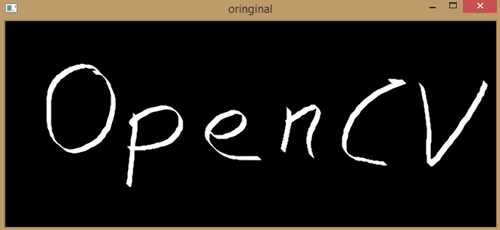#Erosion
#let's define our kernel size

`kernel=np.ones((5,5),np.uint8)`

#now we erode the image, here iteration is no of times you want to erode the image

```erosion=cv2.erode(image,kernel,iterations=1)
cv2.imshow('Erosion’, erosion)
cv2.waitKey(0)

```

#dilation

```dilation=cv2.dilate(image,kernel,iterations=1)
cv2.imshow('dilation',dilation)
cv2.waitKey(0)```

#opening, Good for removing the noise

```opening = cv2.morphologyEx(image,cv2.MORPH_OPEN,kernel)
cv2.imshow('opening',opening)
cv2.waitKey(0)```

#closing, Good for removing noise

```closing=cv2.morphologyEx(image,cv2.MORPH_CLOSE,kernel)
cv2.imshow('closing',closing)
cv2.waitKey(0)
cv2.destroyAllWindows()                                                                ```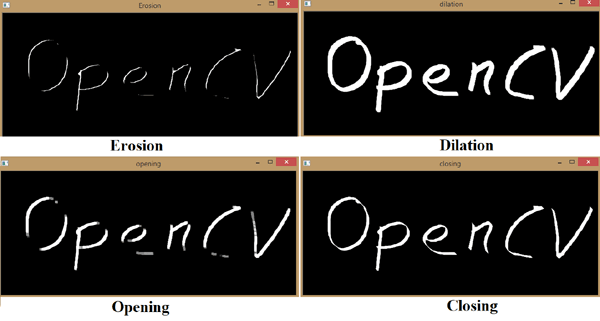### 6. Edge detection and Image gradients

Edge detection is very important area in computer vision, especially when dealing with contours.

Edges can be defined as boundaries of image, actually they are edges which define object in images they preserve a lot of information about the image.

Formally Edges can be defined as sudden changes (discontinuities) in an image and they can encode as much information as pixels.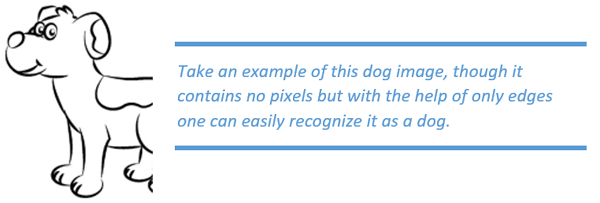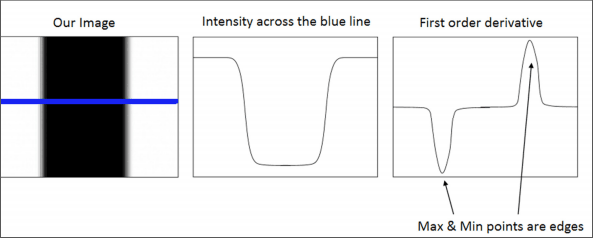The above image shows how computer vision identifies and recognizes the image.

Edge detection algorithms:- There are three main types of edge detection algorithms

• Sobel – to emphasis on vertical or horizontal images.
• Laplacian – optimal due to low error rate, well defined edges and accurate detection.
• Canny Edge detection algorithm (devolped by john.F.Canny in 1986)

1. Applies Gaussian blur

2. Finds intensity gradient of image

3. applies non-maximum suppression (i.e. removes pixels that are not edges).

4. Hysteresis applies threshold (i.e. if pixel is within the upper and lower threshold, it is considered as an edge)

```import cv2
import numpy as np
height,width=image.shape[:2]```

#sobel

#extracting sobel edges

```sobel_x=cv2.Sobel(image,cv2.CV_64F,0,1,ksize=5)
sobel_y=cv2.Sobel(image,cv2.CV_64F,1,0,ksize=5)
cv2.imshow('original',image)
cv2.waitKey(0)
cv2.imshow('sobelx',sobel_x)
cv2.waitKey(0)```

#Sobely

```cv2.imshow('sobely',sobel_y)
cv2.waitKey(0)```

```sobel_OR=cv2.bitwise_or(sobel_x,sobel_y)
cv2.imshow('sobelOR',sobel_OR)
cv2.waitKey(0)```

#laplaian

```laplacian=cv2.Laplacian(image,cv2.CV_64F)
cv2.imshow('Laplacian',laplacian)
cv2.waitKey(0)```

#canny edge detection algorithm uses gradient values as thresholds
#in canny we need to provide two values: threshold1 and threshold2.
#any gradient larger than threshold 2 is considered to be an edge.
#any gradient larger than threshold 1 is considered not to be an edge.
#values in between threshold 1 and threshold 2 are either as edge or non-edge
#on how their intensities are connected, in this case any value below 60 are considered
#non edges wheareas any value above 120 are considered as edges.

```canny=cv2.Canny(image,60,120)
cv2.imshow('canny',canny)
cv2.waitKey(0)
cv2.destroyAllWindows()```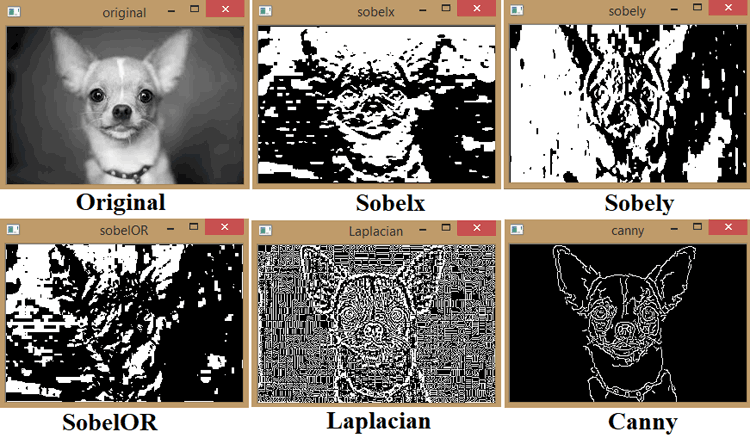### 14. Perspective & Affine Transform

Let’s take a step back and have a look at affine and non-affine transforms, the original image shown below is clearly an non affine image as the edges are going to meet at some point however, we can straighten it by warping and taking the perspective transform.

For this perspective transform we need the four coordinates of the original image and then the four points of the output image, they are denoted by points_A and points_B. Firstly with the help of these points we compute a transformation matrix, M with the help of getPerspectiveTransform function.

And then this matrix is given to the warpPerspective function to generate the final output.

Now let’s first try the Perspective transform.

```import cv2
import numpy as np
import matplotlib.pyplot as plt
cv2.imshow('original',image)
cv2.waitKey(0)```

#coordinate of 4 corners of original image

`points_A=np.float32([[320,15],[700,215],[85,610],[530,780]])`

#coordinates of 4 corners of desired output
#we use a ratio of an A4 paper 1:1.41

`points_B=np.float32([[0,0],[420,0],[0,592],[420,592]])`

#use the two sets of two points to compute the prespective transformation matrix,M

```M=cv2.getPerspectiveTransform(points_A,points_B)
warped=cv2.warpPerspective(image,M,(420,594))
cv2.imshow('warpprespective',warped)
cv2.waitKey(0)
cv2.destroyAllWindows()```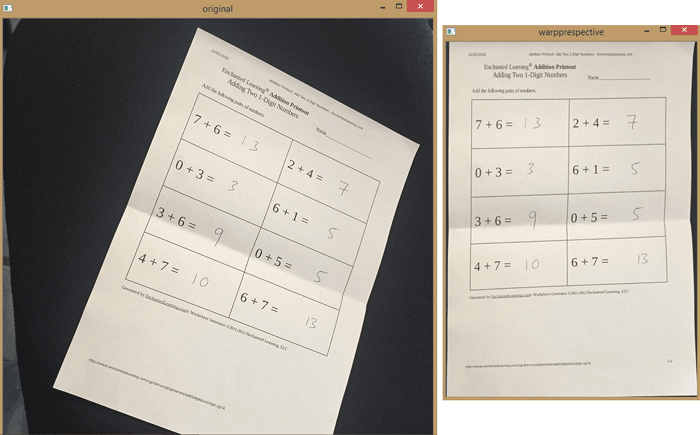Affine transform is easier then the non-affine transform as we need only three points to get the transform. The whole process goes the same but instead of perspective transform we now have affine transform and also we define cols and rows in warpAffine from the shape function instead of manually entering it.

Now let’s try out the affine transform

```import cv2
import numpy as np
import matplotlib.pyplot as plt
rows, cols=image.shape[:2]
cv2.imshow('original',image)
cv2.waitKey(0)```

#coordinate of 3 corners of original image

`points_A=np.float32([[320,15],[700,215],[85,610]])`

#coordinates of 3 corners of desired output
#we use a ratio of an A4 paper 1:1.41

`points_B=np.float32([[0,0],[420,0],[0,592]])`

#use the two sets of two points to compute the Affine
#transformation matrix,M

```M=cv2.getAffineTransform(points_A,points_B)
warped=cv2.warpAffine(image,M,(cols,rows))
cv2.imshow('warpaffine',warped)
cv2.waitKey(0)
cv2.destroyAllWindows()```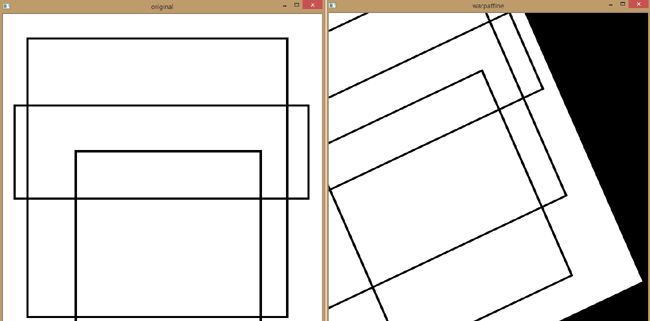### 8. Live Sketch Application

First of all, congratulate yourself that you have made up to this mini project after reading all the image manipulation functions above. So in this mini project of Python OpenCV we are going to learn some new concepts of loops and functions. If you are familiar with programming, you must have a broader idea of what the function and loops are. However, in python the basic concept of loops and functions remains the same but the method to define them changes a little.

So at start of this program we can see a certain group of statements heading under the “def sketch(image) :” this is a formal definition of a function a group of statements working together for a certain output.

So this sketch is a function, in python function is defined by “def” and ends with a “:” mark. Also the statements which are required to be inside the function or you can say which are required for the function to work properly, are side aligned automatically by the function. So to come out of the functions the statements needed to be totally left aligned. For the further references you can refer to google on how the functions are defined in python.

So in this sketch function we have introduced several layers of image processing which combine together to give an output. Firstly, the image is converted into grayscale so the opencv can process it easily and then a Gaussian blur is applied to the gray scale image so as to reduce the noise. Then the edges are being extracted with the help of the canny’s edge detection algorithm then a binary inverse is applied on the edge defined image, here the binary inverse could also be done by bitwise_NOT but we had deliberately chosen this threshold binary inverse as it gives freedom to sets its parameters till we get a clear image.

Also to note that the function takes the arguments image and returns the two arguments ret and mask. While the ret is the Boolean telling that function is run successfully or not and the mask is the final output of the function i.e. the processed image.

Then the second concept is of operating webcam in opencv that is done by cv2.VideoCapture(0) function, which stores the image in an object cap that cap can be read with cap.read() function, also here to note that cap.read() is inside the infinite while loop as it continuously had to capture the images, to give it a sense of a live video, where the frame rate of the video would be the frame rate of your webcam which is mostly between 24 to 60 fps.

cap.read() returns ret and frame, where the ret is the Boolean indicating that the function was successfully run or not and the frame contains the image taken by webcam.

Below is the complete Python OpenCV code for running the Live Sketch

```import cv2
import numpy as np

#sketch generating function
def sketch(image):
#convert image to grayscale
img_gray=cv2.cvtColor(image,cv2.COLOR_BGR2GRAY)

#cleaning up the image using Gaussian blur
img_gray_blur=cv2.GaussianBlur(img_gray,(5,5),0)

#extract edges

canny_edges=cv2.Canny(img_gray_blur,10,70)

#do an invert binarize the image

#initialize webcam, cap is the object provided by video capture
#it contains a Boolean indicating if it was successful(ret)
#it also contains the images collected from the webcam(frame)

cap=cv2.VideoCapture(0)
while True:
cv2.imshow('livesketcher',sketch(frame))
if cv2.waitKey(1) == 13:    #13 is the enterkey
break

#release camera and close window, remember to release the webcam with the help of cap.release()
cap.release()
cv2.destroyAllWindows()```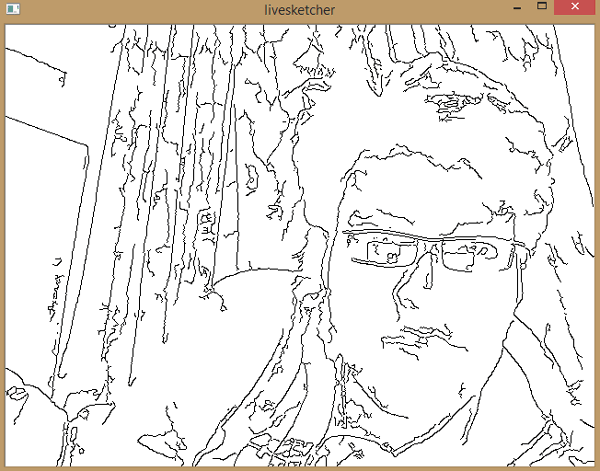So this is the end of Part 2 of Image manipulations in Python-OpenCV. To get good understating of computer vision and OpenCV, go through previous articles (Getting started with Python OpenCV and Image Manipulations in Python OpenCV (Part 1) and you will be able to make something cool with Computer Vision.